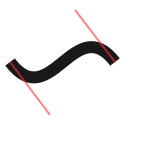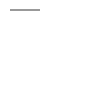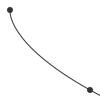# Paths¶

`beginpath`(x=None, y=None)

Start a new Bézier path. This command is needed before any other path drawing commands such as `moveto()`, `lineto()`, or `curveto()`. Finally, the `endpath()` command draws the path on the screen.

If x and y are not specified, this command should be followed by a `moveto()` call.

`moveto`(x, y)

Move the Bézier “pen” to the specified point without drawing.

Can only be called between `beginpath()` and `endpath()`.

`lineto`(x, y)

Draw a line from the pen’s current point to the specified (x,y) coordinates.

Can only be called between `beginpath()` and `endpath()`.

`curveto`(x1, y1, x2, y2, x3, y3)

Draws a curve between the current point in the path and a new destination point.

The last two parameters are the coordinates of the destination point. The first 4 parameters are the coordinates of the two control points, which define the edge and slant of the curve.

Can only be called between `beginpath()` and `endpath()`.```x, y = 10, 62     # Start curve point
x1, y1 = 50, 115  # Left control point
x2, y2 = 75, 10   # Right control point
x3, y3 = 115, 62  # End curve point

# Only strokes
autoclosepath(False)
nofill()
strokewidth(12)
stroke(0.1)

# Draw the curve
beginpath()
moveto(x, y)
curveto(x1, y1, x2, y2, x3, y3)
endpath()

# To show where the control points are,
# we draw helper lines
strokewidth(2)
stroke(1, 0.2, 0.2, 0.6)
# The first control point starts at the
# x, y position
line(x, y, x1, y1)
# And the second control point is the
# end curve point
line(x2, y2, x3, y3)
```
`arcto`(x, y, radius, angle1, angle2)

Continues the path with a circular arc in a way identical to `arc()`. A line will be drawn between the current point and the arc’s starting point.

`closepath`()

Close the path; in case the current point is not the path’s starting point, a line will be drawn between them.

`endpath`(draw=True)

The endpath() command is the companion command to beginpath(). When endpath() is called, the path defined between beginpath() and endpath() is drawn. Optionally, when endpath(draw=False) is called, the path is not drawn but can be assigned to a variable and drawn to the screen at a later time with the drawpath() command.

`drawpath`(path)

Draws a path on the screen. A path is a series of lines and curves defined between beginpath() and endpath(). Normally, endpath() draws the path to the screen, unless when calling endpath(draw=False). The path can then be assigned to a variable, and this variable used as a parameter for drawpath().

Note: if you have one path that you want to draw multiple times with drawpath(), for example each with its own rotation and position, you need to supply a copy with `drawpath(path.copy())`.```stroke(0.2)
beginpath(10, 10)
lineto(40, 10)
p = endpath(draw=False)
drawpath(p)
```
`autoclosepath`(close=True)

Defines whether paths are automatically closed by connecting the last and first points with a line. It takes a single parameter of True or False. All shapes created with beginpath() following this command will adhere to the setting.

`findpath`(points, curvature=1.0)

Constructs a fluid path from a list of coordinates. Each element in the list is a 2-tuple defining the x-coordinate and the y-coordinate. If the curve has more than three points, the curvature parameter offers some control on how separate segments are stitched together: from straight lines (0.0) to smooth curves (1.0).```points = [(10, 10), (90, 90), (350, 200)]
ellipsemode(CENTER)
for x, y in points:
ellipse(x, y, 6, 6)

nofill()
stroke(0.2)
autoclosepath(False)
path = findpath(points)
drawpath(path)
```
`beginclip`(path)
`endclip`()

The beginclip() and endclip() commands define a clipping mask. The supplied parameter defines the path to be used as a clipping mask.

All basic shapes and path commands return paths that can be used with beginclip() - setting the `draw` parameter of a shape command will simply return the path without actually drawing the shape. Any shapes, paths, texts and images between beginclip() and endclip() are clipped: any part that falls outside the clipping mask path is not drawn.```p = ellipse(20, 20, 60, 60, draw=False)
beginclip(p)
stroke(0.5)
strokewidth(15)
line(20, 20, 80, 80)
line(20, 80, 80, 20)
line(50, 20, 50, 80)
endclip()
```